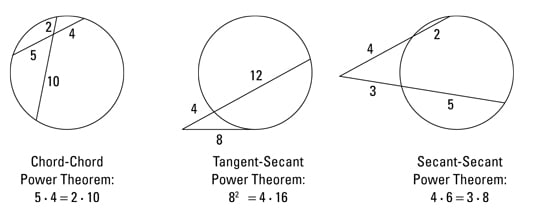##### Geometry Essentials For Dummies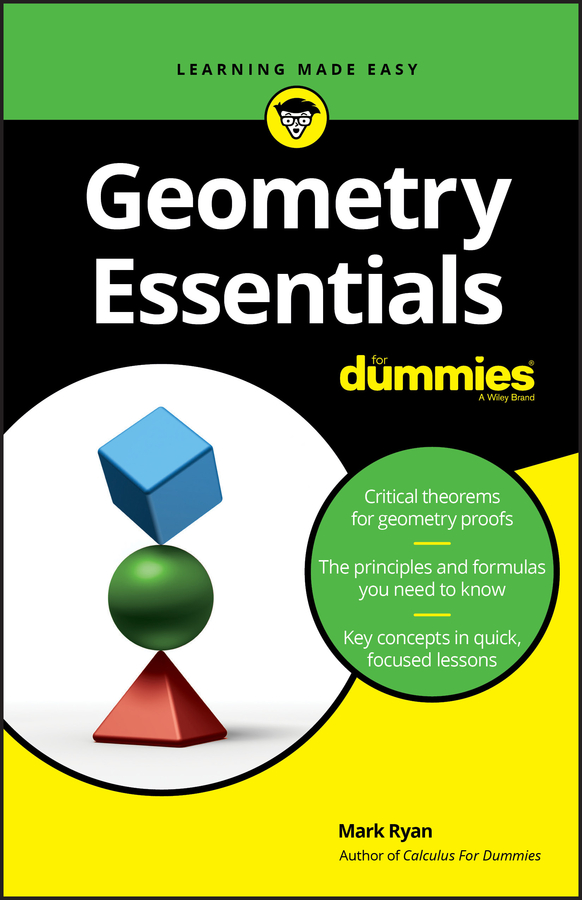Below are several of the most important geometry formulas, theorems, properties, and so on that you use for solving various problems. If you get stumped while working on a problem and can’t come up with a formula, this is the place to look.

## Triangle Formulas

• Sum of the interior angles of a triangle: 180°

• Area: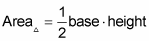• Hero’s area formula: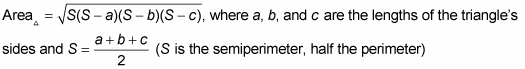• Area of an equilateral triangle: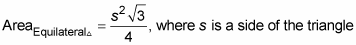• The Pythagorean Theorem: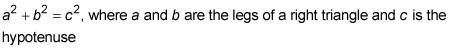• Common Pythagorean triples (side lengths in right triangles):

• 3-4-5

• 5-12-13

• 7-24-25

• 8-15-17

• Ratios of the sides in special right triangles:

• The sides opposite the angles in a 45°- 45°- 90° triangle are in the ratio of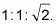• The sides opposite the angles in a 30°- 60°- 90° triangle are in the ratio of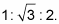• Altitude-on-Hypotenuse Theorem: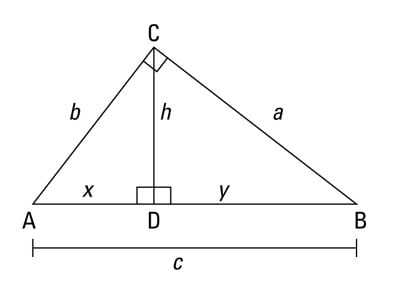If an altitude is drawn to the hypotenuse of a right triangle as shown in the above figure, then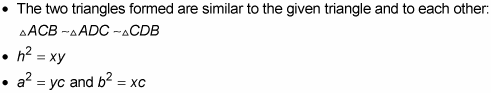## Polygon Formulas

• Area formulas:

• Parallelogram: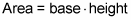• Rectangle:• Kite or rhombus: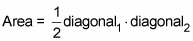• Square: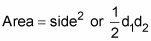• Trapezoid: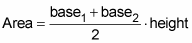• Regular polygon: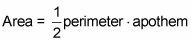• Sum of the interior angles in an n-sided polygon: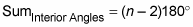• Measure of each interior angle of a regular (or other equiangular) n-sided polygon: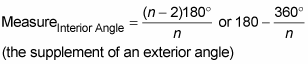• Sum of the exterior angles (one at each vertex) of any polygon: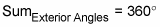• Measure of each exterior angle of a regular (or other equiangular) n-sided polygon: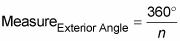• Number of diagonals that can be drawn in an n-sided polygon: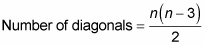## Circle Formulas

• Circumference: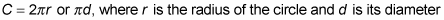• Area: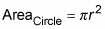• Arc length: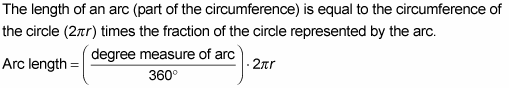• Sector area: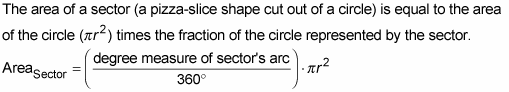• Measure of an angle . . .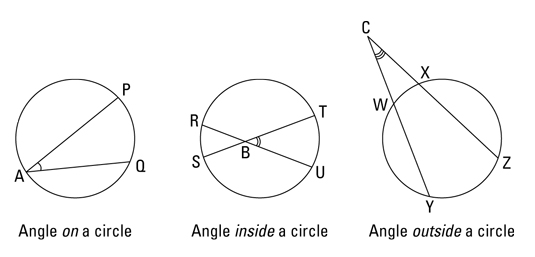• On a circle: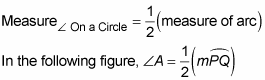• Inside a circle: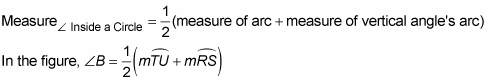• Outside a circle: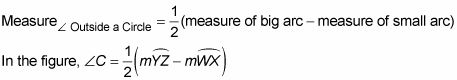• Chord-Chord Power Theorem: When two chords of a circle intersect, the product of the parts of one chord is equal to the product of the parts of the other chord.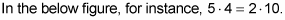• Tangent-Secant Power Theorem: When a tangent and a secant of a circle meet at an external point, the measure of the tangent squared is equal to the product of the secant’s external part and its total length.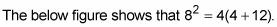• Secant-Secant Power Theorem: When two secants of a circle meet at an external point, the product of one secant’s external part and its total length is equal to the product of the other secant’s external part and its total length.

In the below figure, 4(4 + 2) = 3(3 + 5).